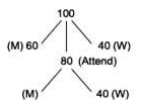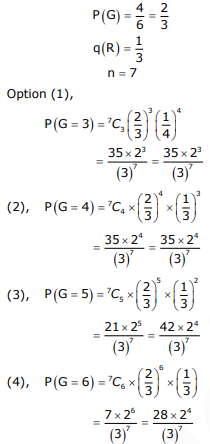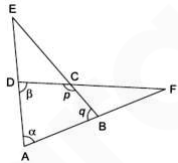# GATE Solved Paper 2017-19 - GATE 2018

• A

2 : 3• B

1 : 1• C

3 : 2• D

2 : 1• Option : B
• Explanation :
Let the total number of people are 100.So, M must be 80 – 40 = 40
Ratio of male of female
40 : 40
1 : 1

• A

Three green faces and four red faces.• B

Four green faces and three red faces.• C

Five green faces and two red faces.• D

Six green faces and one red face.• Option : C
• Explanation :
Four green, two red faceOption 3 is maximum value.
So, five green faces and two red faces.

• A

∠ BCD – ∠ BAD• B

∠ BAD + ∠ BCF• C

∠ BAD + ∠ BCD• D

∠ CBA + ∠ ADC• Option : A
• Explanation :∠E + ∠F = ?
α + q + E = 180       ...(i)
α + β + F = 180       ...(ii)
α + β + p + q = 360       ...(iii)
Equation (i) + (ii) = (iii)
α + q + E + α + β + F = α + β + p + q
E + F = p - α

• A

1,50,000• B

2,00,000• C

1,75,000• D

1,51,000• Option : B
• Explanation :
Male + Female = 300     ...(i)
Total money = (8/9)M x 750 + (2/3)F x 1000
= (6000/9)M + (6000/9) F
= (6000/9)(M + F)
From equation (i)
Total money = (6000/9)(300) = 2,00,000

• A

-1• B

1/pqr• C

1• D

pqr• Option : C
• Explanation :
px = q
qy = r
xlogp = logq
ylogq = logr
zlogr = logp
x = logq/yp
y = yz/logq
z = logp /yr
x x y x z = 1
Related Quiz.
GATE 2018